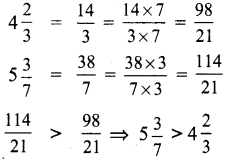## Tamilnadu Samacheer Kalvi 6th Maths Solutions Term 3 Chapter 1 Fractions Additional Questions

Question 1.
Color the part according to the given fraction.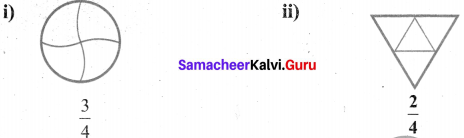Solution:
(i) Here $$\frac{3}{4}$$ shows out of 4 parts 3 parts are shaded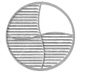(ii) Here $$\frac{2}{4}$$ shows out of 4 parts 2 parts are shaded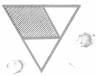Question 2.
Identify the error if any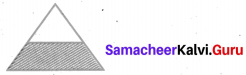Solution:
In the given figure, shaded portion is not equal to unshaded portion. So the fraction is not equal to $$\frac{1}{2}$$.

Question 3.
What fraction of an hour is 20 minutes?
Solution:
We know that total minutes in an hour = 60 min
∴ Required fraction = $$\frac{20 \min }{60 \min }=\frac{20}{60}=\frac{1}{3}$$Question 4.
Write a fraction equivalent to $$\frac{3}{5}$$ with numerator 15.
Solution:
Given, numerator of an equivalent fraction = 15
Equivalent fraction of $$\frac{3}{5}=\frac{3 \times 5}{5 \times 5}=\frac{15}{25}$$

Question 5.
Which is the larger fraction $$\frac{6}{10}$$ or $$\frac{7}{10}$$?
Solution:
Here the denominators, of both fractions are same.
Also 7 > 6 So $$\frac{7}{10}>\frac{6}{10}$$

Question 6.
Sona got one-fifth of the total marks and Mala got one-third of the total marks. Who got more?
Solution:
We know that if the numerators are same in two fractions, the fraction with smaller denominator is greater.
∴ $$\frac{1}{3}>\frac{1}{5}$$
∴ Mala got more marks.

Question 7.
A piece of rope $$\frac{7}{8}$$ metre long is cut into two pieces. One piece was $$\frac{1}{4}$$ m long. How long is the other?
Solution: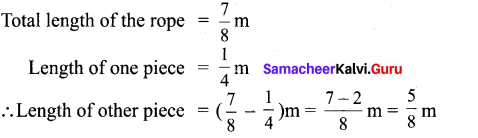Question 8.
Meena travelled 3$$\frac{1}{2}$$ km by bus, then she walked 1$$\frac{1}{8}$$ km to reach a town. How much she travelled to reach-the town?
Solution: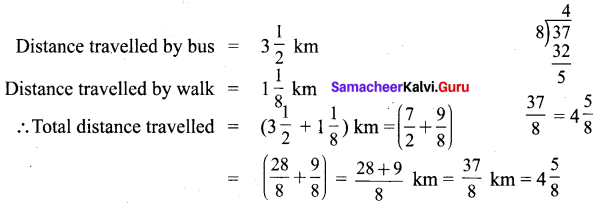She travelled 4$$\frac{5}{8}$$ kmQuestion 9.
What should be subtracted from the sum of 2$$\frac{1}{4}$$ and 3$$\frac{1}{7}$$ to get 2$$\frac{3}{28}$$ ?
Solution: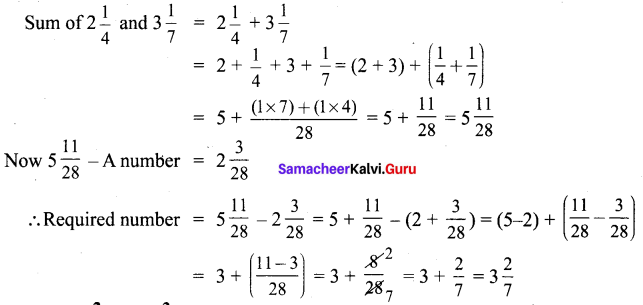Question 10.
Compare 4$$\frac{2}{3}$$ and 5$$\frac{3}{7}$$?
Solution: